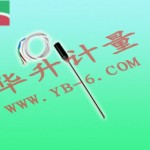•# 温度传感器

• 作者: clcc123
• 日期: 2016-09-09 14:29:51
• 人气: -
• 评论: 0
• 标签: 温度传感器
• 分享到：

温度传感器系列手持式温度传感器适于手持测量现场温度，传感器配有螺纹固定方式，也可以用于各种通过螺纹固定测温的各种场所。

 手柄式温度传感器选型 100型 温度传感器 口 WRN-K  WRE-E 热电偶类型 T口 0=0～400  1=0～800  3=指定 温度范围(℃) 口 A=A级      B=B级 传感器精度 口 1=SUS321   4=SUS304    6=SUS316      9=指定 保护管材质 口 4=4   5=5   6=6   7=7   8=8    9=指定 保护管直径 M口 1=无螺纹连接   2=M8×1      3=M8×1.254=M10×1       5=M10×1.5   6=M12×17=M12×1.5     8=M16×1.5   9=指定 螺纹规格 G口 2=20      3=30    4=40    5=50    6=60   8=80  10=100    20=200   9=指定 探头长度(mm) L口 1=100      2=200     3=300   5=500  10=1000  30=3000    50=5000   9=指定 引线长度(mm) X口 2=两线制  3=三线制  4=四线制 引出线采用线制 口 1=聚氯乙烯PVC（-20～80℃）         2=聚氨酯TPU （-50～100℃） 9=指定 引线材质“（）”内为常用温度 口 0=无屏蔽层（默认）1=有屏蔽层 是否配屏蔽层 口 0=无护套     1=有护套 线缆护套 口 0=无     1=镀锡      3=压M4端子   9=指定 导线末端要求 Q 0=无    1=配螺母  9=指定 特殊要求 说明：对于用户指定的参数要求，直接以数字或文字标注出来即可

## 访问排行

Copyright © 2012-2014 金湖县华升计量仪器有限公司 Inc. 保留所有权利。 Powered by TWCMS 2.0.3